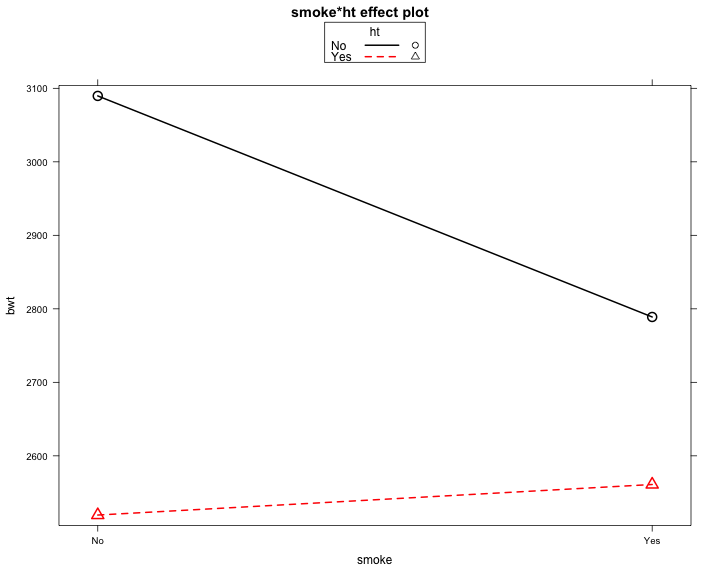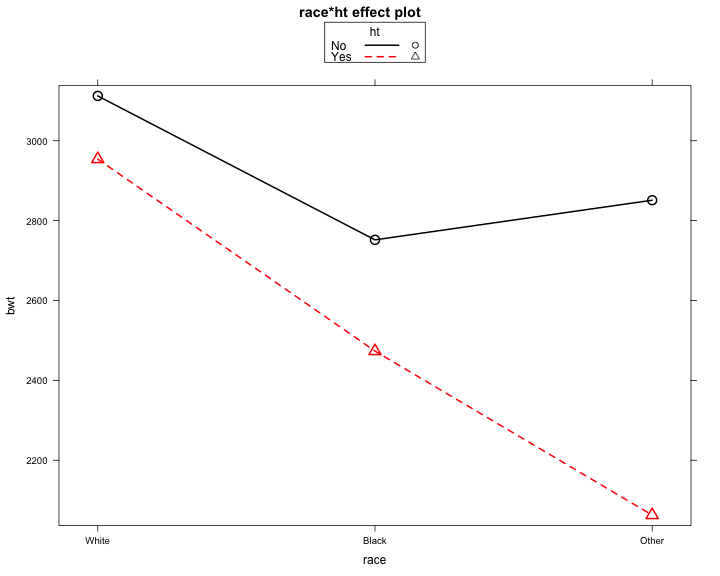# BIO 213 Regression: Interaction between two categorical variables

``````## Settings for RMarkdown http://yihui.name/knitr/options#chunk_options
opts_chunk\$set(comment = "", warning = FALSE, message = FALSE, tidy = FALSE,
echo = T, fig.width = 10, fig.height = 8)
options(width = 125, scipen = 5, digits = 5)

setwd("~/statistics/bio213/")
``````

``````library(gdata)

## Recoding
lbw <- within(lbw, {
## race relabeling
race <- factor(race, levels = 1:3, labels = c("White","Black","Other"))

## ftv (frequency of visit) relabeling
ftv.cat <- cut(ftv, breaks = c(-Inf, 0, 2, Inf), labels = c("None","Normal","Many"))
ftv.cat <- relevel(ftv.cat, ref = "Normal")

## ptl
preterm <- factor(ptl >= 1, levels = c(F,T), labels = c("=0",">=1"))
})

no.yes.variables <- c("ht","ui","smoke","low")

lbw[,no.yes.variables] <-
lapply(lbw[,no.yes.variables],
function(var) {
var <- factor(var, levels = 0:1, labels = c("No","Yes"))
})

## Same data available online: http://www.umass.edu/statdata/statdata/data/index.html
## Cases 10 and 39 needs fix to make them identical to Dr. Orav's dataset
## lbw2[c(10,39),"BWT"] <- c(2655,3035)
``````

## Tabulation of means in subgroups: smoke * ht interaction

``````## Using xtabs
tab.means.by.smoke.ht <- xtabs(bwt ~ smoke +ht, lbw) / xtabs( ~ smoke +ht, lbw)
tab.means.by.smoke.ht
``````
``````     ht
smoke     No    Yes
No  3089.7 2519.4
Yes 2788.9 2561.0
``````
``````
## Using Hmisc package
library(Hmisc)
summary(bwt ~ smoke + ht, data = lbw, method = "cross", fun = mean)
``````
``````
UseMethod by smoke, ht

+---+
|N  |
|bwt|
+---+
+-----+------+------+------+
|smoke|  No  |  Yes |  ALL |
+-----+------+------+------+
| No  |108   |  7   |115   |
|     |3089.7|2519.4|3055.0|
+-----+------+------+------+
| Yes | 69   |  5   | 74   |
|     |2788.9|2561.0|2773.5|
+-----+------+------+------+
| ALL |177   | 12   |189   |
|     |2972.4|2536.8|2944.8|
+-----+------+------+------+
``````

## Fit models: smoke * ht interaction

The model means:

``````- mean bwt in the smoke No , ht No  group is 3089.67
- mean bwt in the smoke Yes, ht No  group is 3089.67 - 300.75
- mean bwt in the smoke No , ht Yes group is 3089.67          - 570.24
- mean bwt in the smoke Yes, ht Yes group is 3089.67 - 300.75 - 570.24 + 342.33
``````
``````model.smoke.ht.interaction <- lm(bwt ~ smoke + ht + smoke:ht, lbw)
summary(model.smoke.ht.interaction)
``````
``````
Call:
lm(formula = bwt ~ smoke + ht + smoke:ht, data = lbw)

Residuals:
Min      1Q  Median      3Q     Max
-2079.9  -452.7    14.3   524.3  1900.3

Coefficients:
Estimate Std. Error t value Pr(>|t|)
(Intercept)      3089.7       68.6   45.05   <2e-16 ***
smokeYes         -300.8      109.8   -2.74   0.0068 **
htYes            -570.2      278.0   -2.05   0.0416 *
smokeYes:htYes    342.3      431.6    0.79   0.4287
---
Signif. codes:  0 '***' 0.001 '**' 0.01 '*' 0.05 '.' 0.1 ' ' 1

Residual standard error: 713 on 185 degrees of freedom
Multiple R-squared: 0.0595, Adjusted R-squared: 0.0443
F-statistic:  3.9 on 3 and 185 DF,  p-value: 0.00984

``````

## Interaction plot: smoke * ht interaction

Non-parallel lines indicate interaction.

``````library(effects)
plot(effect("smoke:ht", model.smoke.ht.interaction), multiline = TRUE)
``````## Tabulation of means in subgroups: race * ht interaction

``````## Using xtabs
tab.means.by.race.ht <- xtabs(bwt ~ race +ht, lbw) / xtabs( ~ race +ht, lbw)
tab.means.by.race.ht
``````
``````       ht
race        No    Yes
White 3112.2 2954.0
Black 2751.8 2473.3
Other 2851.1 2062.8
``````
``````
## Using Hmisc package
library(Hmisc)
summary(bwt ~ race + ht, data = lbw, method = "cross", fun = mean)
``````
``````
UseMethod by race, ht

+---+
|N  |
|bwt|
+---+
+-----+------+------+------+
| race|  No  |  Yes |  ALL |
+-----+------+------+------+
|White| 91   |  5   | 96   |
|     |3112.2|2954.0|3103.9|
+-----+------+------+------+
|Black| 23   |  3   | 26   |
|     |2751.8|2473.3|2719.7|
+-----+------+------+------+
|Other| 63   |  4   | 67   |
|     |2851.1|2062.8|2804.0|
+-----+------+------+------+
|ALL  |177   | 12   |189   |
|     |2972.4|2536.8|2944.8|
+-----+------+------+------+
``````

## Fit models: smoke * ht interaction

The model means:

``````                                           intercept   raceB   raceO   htYes  rB:htY  rO:htY
- mean bwt in the race White, ht No  group is 3112.2
- mean bwt in the race Black, ht No  group is 3112.2 - 360.4
- mean bwt in the race Other, ht No  group is 3112.2         - 261.1
- mean bwt in the race White, ht Yes group is 3112.2                 - 158.2
- mean bwt in the race Black, ht Yes group is 3112.2 - 360.4         - 158.2 - 120.3
- mean bwt in the race Other, ht Yes group is 3112.2         - 261.1 - 158.2         - 630.1
``````
``````model.race.ht.interaction <- lm(bwt ~ race + ht + race:ht, lbw)
summary(model.race.ht.interaction)
``````
``````
Call:
lm(formula = bwt ~ race + ht + race:ht, data = lbw)

Residuals:
Min      1Q  Median      3Q     Max
-2142.1  -475.2    11.9   530.8  1877.8

Coefficients:
Estimate Std. Error t value Pr(>|t|)
(Intercept)       3112.2       74.4   41.83   <2e-16 ***
raceBlack         -360.4      165.6   -2.18    0.031 *
raceOther         -261.1      116.3   -2.24    0.026 *
htYes             -158.2      326.0   -0.49    0.628
raceBlack:htYes   -120.3      544.2   -0.22    0.825
raceOther:htYes   -630.1      490.1   -1.29    0.200
---
Signif. codes:  0 '***' 0.001 '**' 0.01 '*' 0.05 '.' 0.1 ' ' 1

Residual standard error: 710 on 183 degrees of freedom
Multiple R-squared: 0.0774, Adjusted R-squared: 0.0522
F-statistic: 3.07 on 5 and 183 DF,  p-value: 0.0109

``````

## Interaction plot: race * ht interaction

Non-parallel lines indicate interaction.

``````library(effects)
plot(effect("race:ht", model.race.ht.interaction), multiline = TRUE)
``````## Relevel to test for diffrences between different groups

No p-values are avalilable for a diffence that is a sum of a main effect and an interaction effect. To get it, change the reference levels so that the difference of interest becomes a simple main effect.

``````lbw.relevel <- lbw
``````

Hypertensive as a reference ht category

Now the coefficients for raceBlack and raceOther are differences between Black and White and Other and White among the hypertensive patients.

``````lbw.relevel\$ht <- relevel(lbw.relevel\$ht, ref = "Yes")
model.race.ht.interaction <- lm(bwt ~ race + ht + race:ht, lbw.relevel)
summary(model.race.ht.interaction)
``````
``````
Call:
lm(formula = bwt ~ race + ht + race:ht, data = lbw.relevel)

Residuals:
Min      1Q  Median      3Q     Max
-2142.1  -475.2    11.9   530.8  1877.8

Coefficients:
Estimate Std. Error t value Pr(>|t|)
(Intercept)        2954        317    9.31   <2e-16 ***
raceBlack          -481        518   -0.93    0.355
raceOther          -891        476   -1.87    0.063 .
htNo                158        326    0.49    0.628
raceBlack:htNo      120        544    0.22    0.825
raceOther:htNo      630        490    1.29    0.200
---
Signif. codes:  0 '***' 0.001 '**' 0.01 '*' 0.05 '.' 0.1 ' ' 1

Residual standard error: 710 on 183 degrees of freedom
Multiple R-squared: 0.0774, Adjusted R-squared: 0.0522
F-statistic: 3.07 on 5 and 183 DF,  p-value: 0.0109

``````
``````
## Reference back to ht = No
lbw.relevel\$ht <- relevel(lbw.relevel\$ht, ref = "No")
``````

Black as a reference race category

Now the coefficient for htYes represents the effect of hypertension in Blacks.

``````lbw.relevel\$race <- relevel(lbw.relevel\$race, ref = "Black")
model.race.ht.interaction <- lm(bwt ~ race + ht + race:ht, lbw.relevel)
summary(model.race.ht.interaction)
``````
``````
Call:
lm(formula = bwt ~ race + ht + race:ht, data = lbw.relevel)

Residuals:
Min      1Q  Median      3Q     Max
-2142.1  -475.2    11.9   530.8  1877.8

Coefficients:
Estimate Std. Error t value Pr(>|t|)
(Intercept)       2751.8      148.0   18.59   <2e-16 ***
raceWhite          360.4      165.6    2.18    0.031 *
raceOther           99.3      172.9    0.57    0.567
htYes             -278.5      435.7   -0.64    0.523
raceWhite:htYes    120.3      544.2    0.22    0.825
raceOther:htYes   -509.8      569.0   -0.90    0.371
---
Signif. codes:  0 '***' 0.001 '**' 0.01 '*' 0.05 '.' 0.1 ' ' 1

Residual standard error: 710 on 183 degrees of freedom
Multiple R-squared: 0.0774, Adjusted R-squared: 0.0522
F-statistic: 3.07 on 5 and 183 DF,  p-value: 0.0109

``````

Other as a reference race category

Now the coefficient for htYes represents the effect of hypertension in Others.

``````lbw.relevel\$race <- relevel(lbw.relevel\$race, ref = "Other")
model.race.ht.interaction <- lm(bwt ~ race + ht + race:ht, lbw.relevel)
summary(model.race.ht.interaction)
``````
``````
Call:
lm(formula = bwt ~ race + ht + race:ht, data = lbw.relevel)

Residuals:
Min      1Q  Median      3Q     Max
-2142.1  -475.2    11.9   530.8  1877.8

Coefficients:
Estimate Std. Error t value Pr(>|t|)
(Intercept)       2851.1       89.4   31.88   <2e-16 ***
raceBlack          -99.3      172.9   -0.57    0.567
raceWhite          261.1      116.3    2.24    0.026 *
htYes             -788.3      366.0   -2.15    0.033 *
raceBlack:htYes    509.8      569.0    0.90    0.371
raceWhite:htYes    630.1      490.1    1.29    0.200
---
Signif. codes:  0 '***' 0.001 '**' 0.01 '*' 0.05 '.' 0.1 ' ' 1

Residual standard error: 710 on 183 degrees of freedom
Multiple R-squared: 0.0774, Adjusted R-squared: 0.0522
F-statistic: 3.07 on 5 and 183 DF,  p-value: 0.0109

``````